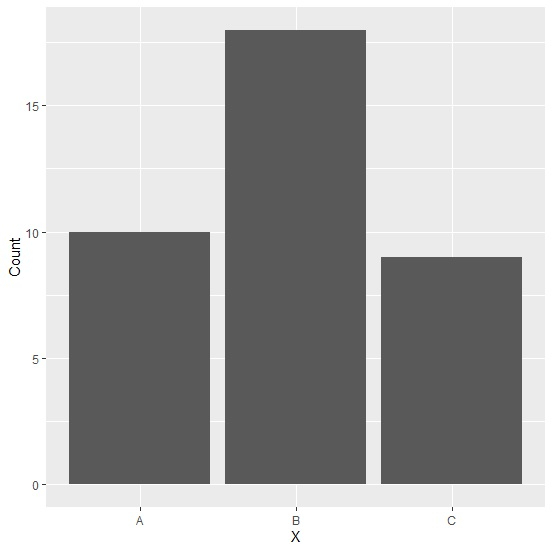# How to create bar plot using ggplot2 with structure data frame?

To create bar plot using ggplot2 with structure data, we need to set the stat argument of geom_bar function to identity. This is same for the regular data frame.

For example, if we have a structured data frame called df that contains a column X for categories and a numerical column say Y for frequency then we can the bar plot for this data by using the below given command −

ggplot(df,aes(X,Y))+geom_bar(stat="identity")

## Example

Following snippet creates a sample data frame −

df<-structure(list(X=c("A","B","C"),Count=c(10,18,9)),.Names=c("X","Count"),row.names=c(NA, -3L),class="data.frame")
df

## Output

The following dataframe is created −

   X  Count
1  A  10
2  B  18
3  C   9

To load ggplot2 package and create bar plot for data in df, add the following code to the above snippet −

library(ggplot2)
ggplot(df,aes(x=X,y=Count))+geom_bar()

## Output

If you execute all the above given snippets as a single program, it generates the following Output −

Error: stat_count() can only have an x or y aesthetic.
Run rlang::last_error() to see where the error occurred.

The correct code to load ggplot2 package and create bar plot is as follows −

ggplot(df,aes(x=X,y=Count))+geom_bar(stat="identity")


## Output

If you execute all the above given snippets as a single program, it generates the following Output −